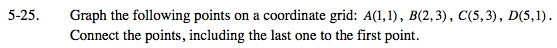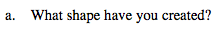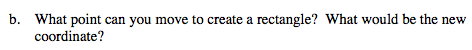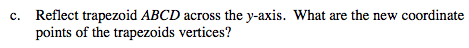### Home > MC2 > Chapter 5 > Lesson 5.1.2 > Problem5-25

5-25.
1. Graph the following points on a coordinate grid: A(1, 1), B(2, 3), C(5, 3), D (5, 1). Connect the points, including the last one to the first point. 5-25 HW eTool (Desmos). Homework Help ✎

1. What shape have you created?

2. What point can you move to create a rectangle? What would be the new coordinate?

3. Reflect trapezoid ABCD across the y-axis. What are the new coordinate points of the trapezoids vertices?The shape has four sides and only one pair of parallel sides.Move only one point to a coordinate that would make the shape a rectangle.Explore the problem with the eTool below.
Click the link at right for the full version of the eTool: MC2 5-25 HW eTool